Tags: Rotary, Encoder, Module, Arduino, Projects

# Rotary Encoder Module for Arduino Projects

• 9.00 AED

15.00 AED

• Ex Tax:9.00 AED
• Brands techmaze
• Product Code: Digital
• Availability: In Stock
A rotary encoder is a type of position. sensor which is used for determining the angular position of a rotating shaft. it generates an electrical signal either analog or digital according to the rotation movement.360 degree rotation.Rotation of the rotary encoder may be..
rotary encoder is a type of position. sensor which is used for determining the angular position of a rotating shaft. it generates an electrical signal either analog or digital according to the rotation movement.

• 360 degree rotation.
• Rotation of the rotary encoder may be counted by the positive rotation direction and a reverse direction pulse frequency output during rotation potentiometer not count this rotation count is not limited.
• With buttons on the rotary encoder can be reset to the initial state, that starts counting from 0.
• Operating voltage is 5V with one circle number of pulse 20.
• It would be a great part for many electronic DIY projects.

# This tutorial will help you learn How Rotary Encoder Works and How To Use It with Arduino: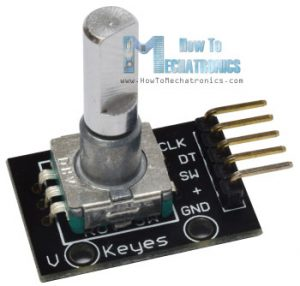There are many different types of rotary encoders which are classified by either Output Signal or Sensing Technology. The particular rotary encoder that we will use in this tutorial is an incremental rotary encoder and it’s the simplest position sensor to measure rotation.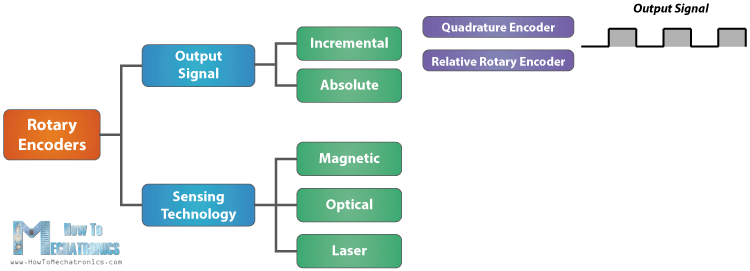This rotary encoder is also known as quadrature encoder or relative rotary encoder and its output is a series of square wave pulses.

## How Rotary Encoder Works

Let’s take a closer look at the encoder and see its working principle. Here’s how the square wave pulses are generated: The encoder has a disk with evenly spaced contact zones that are connected to the common pin C and two other separate contact pins A and B, as illustrated below.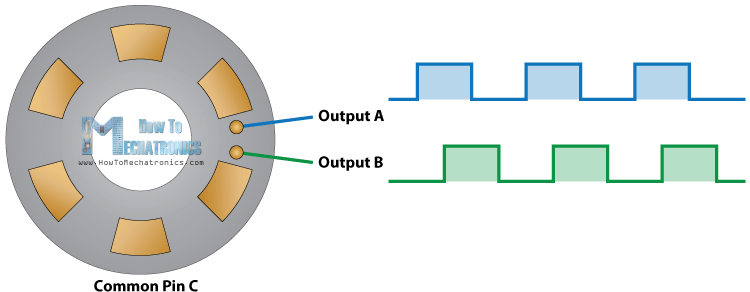When the disk will start rotating step by step, the pins A and B will start making contact with the common pin and the two square wave output signals will be generated accordingly.

Any of the two outputs can be used for determining the rotated position if we just count the pulses of the signal. However, if we want to determine the rotation direction as well, we need to consider both signals at the same time.

We can notice that the two output signals are displaced at 90 degrees out of phase from each other. If the encoder is rotating clockwise the output A will be ahead of output B.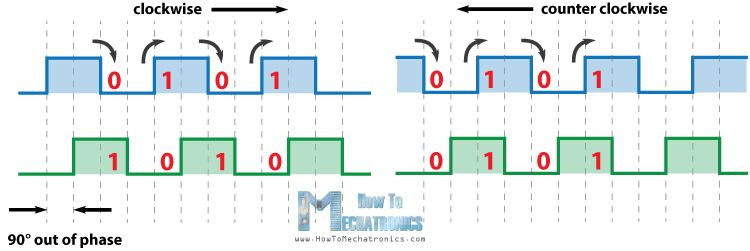So if we count the steps each time the signal changes, from High to Low or from Low to High, we can notice at that time the two output signals have opposite values. Vice versa, if the encoder is rotating counter clockwise, the output signals have equal values. So considering this, we can easily program our controller to read the encoder position and the rotation direction.

## Rotary Encoder Arduino Example

Let’s make a practical example of it using the Arduino. The particular module that I will use for this example comes on a breakout board and it has five pins. The first pin is the output A, the second pin is the output B, the third pin is the Button pin and of course the other two pins are the VCC and the GND pin.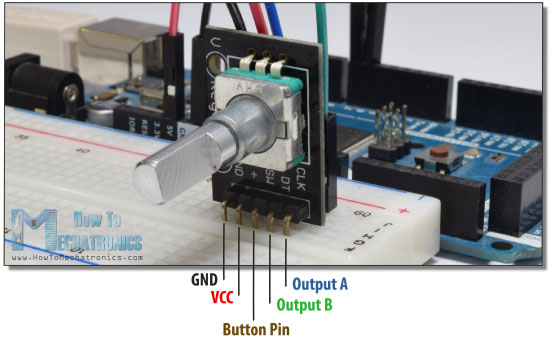We can connect the output pins to any digital pin of the Arduino Board.

Source Code

Here’s the Arduino code:

1. /* Arduino Rotary Encoder Tutorial
2. *
3. * by Dejan Nedelkovski, www.HowToMechatronics.com
4. *
5. */
6. #define outputA 6
7. #define outputB 7
8. int counter = 0;
9. int aState;
10. int aLastState;
11. void setup() {
12. pinMode (outputA,INPUT);
13. pinMode (outputB,INPUT);
14. Serial.begin (9600);
15. // Reads the initial state of the outputA
16. aLastState = digitalRead(outputA);
17. }
18. void loop()

There are no reviews for this product.

## FREE RETURNS

*for faulty items and in-store drop off for unused items. T&C applies.